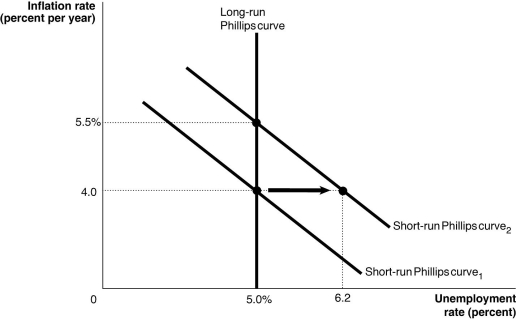# [Solved] Figure 134 Alt Text for Figure 13

## Figure 13.4Alt text for Figure 13.4: In figure 13.4, a graph shows the shift in the short-run Phillips curve.Long description for Figure 13.4: The x-axis is labelled, unemployment rate percent.The y-axis is labelled, inflation rate percent per year.A straight line labelled, short-run Philips Curve 1, begins at the top left corner and slopes down to the end of the x-axis.A straight line labelled, short-run Philips Curve 2, follows the same slope as curve 1, but is plotted to the right.The area between Curve 1 and Curve 2 is indicated by a right pointing arrow.A straight line labelled, long-run Philips Curve 1 is perpendicular to the x-axis, and begins from the x-axis value 5.0%.Long-run Philips Curve 1 intersects the short-run Philips Curve 1 at point (5.0%, 4.0)and intersects the short-run Philips Curve 2 at point (5.0%, 5.5%).Point (6.2, 4.0)is plotted toward the right end of the short-run Philips Curve 2.The points are connected to their respective coordinates on the x-axis and y-axis with dotted lines.-Refer to Figure 13.4.Consider the shift in the short-run Phillips curve shown in the above graph. This shift may be explained by

A)an increase in the natural rate of unemployment from 5.0 to 6.2 percent.
B)an increase in the expected rate of inflation from 4.0 to 5.5 percent.
C)either an increase in the natural rate of unemployment from 5.0 to 6.2 percent or an increase in the expected rate of inflation from 4.0 to 5.5 percent.
D)a decrease in the natural rate of unemployment.
E)none of the above.

Tags

10+ million students use Quizplus to study and prepare for their homework, quizzes and exams through 20m+ questions in 300k quizzes.

Explore our library and get Economics Homework Help with various study sets and a huge amount of quizzes and questions

### Related Questions

Get Free Access Now!